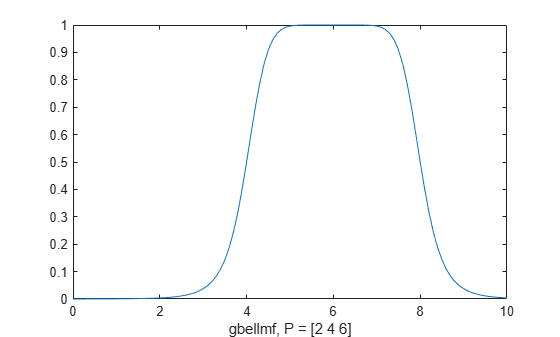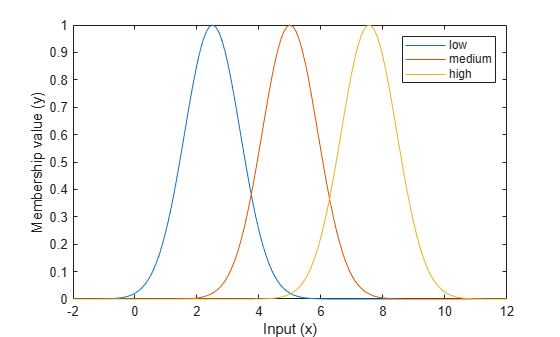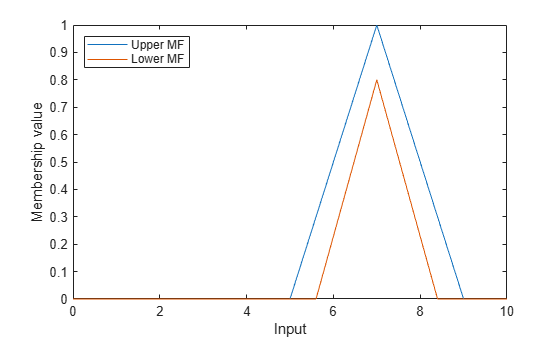# evalmf

Evaluate fuzzy membership function

## Syntax

``y = evalmf(mfT1,x)``
``[yUpper,yLower] = evalmf(mfT2,x)``

## Description

example

````y = evalmf(mfT1,x)` evaluates one or more type-1 membership functions based on the input values in `x`, returning the membership function values.```

example

````[yUpper,yLower] = evalmf(mfT2,x)` evaluates one or more type-2 membership function based on the input values in `x`, returning both the upper and lower membership function values.```

## Examples

collapse all

Evaluate a generalized bell-shaped membership function across a range of input values from `0` through `10`.

```x = 0:0.1:10; mf = fismf("gbellmf",[2 4 6]); y = evalmf(mf,x);```

Plot the evaluation.

```plot(x,y) xlabel('gbellmf, P = [2 4 6]')```Create a vector of three Gaussian membership functions.

```mf = [fismf("gaussmf",[0.9 2.5],'Name',"low"); fismf("gaussmf",[0.9 5],'Name',"medium"); fismf("gaussmf",[0.9 7.55],'Name',"high")];```

Specify the input range over which to evaluate the membership functions.

`x = (-2:0.1:12)';`

Evaluate the membership functions.

`y = evalmf(mf,x);`

Plot the evaluation results.

```plot(x,y) xlabel('Input (x)') ylabel('Membership value (y)') legend("low","medium","high")```Create a triangular type-2 membership function.

`mf = fismftype2("trimf",[5 7 9],'LowerLag',0.3,'LowerScale',0.8);`

Evaluate the membership function across a range of input values from `0` through `10`.

```x = 0:0.1:10; [yUpper,yLower] = evalmf(mf,x);```

Plot the evaluated upper and lower MFs.

```plot(x,yUpper,x,yLower) legend('Upper MF','Lower MF','Location','northwest') xlabel('Input') ylabel('Membership value')```## Input Arguments

collapse all

Type-1 membership function, specified as a `fismf` object or a vector of such objects.

Input value, specified as a scalar, vector, or 2-D matrix. If `mf` is a:

• Single `fismf` object, then you can specify `x` as a scalar, vector, or matrix

• Vector of `fismf` objects, then you can specify `x` as a scalar or vector

Since R2019b

Type-2 membership function, specified as a `fismftype2` object or a vector of such objects.

## Output Arguments

collapse all

Membership value for a type-1 membership function, returned as a scalar, vector, or 2-D matrix. If `mfT1` is a:

• Single `fismf` object, then `y` is a scalar, vector, or matrix with the same dimensions as `x`. Each element of `y` is the evaluated membership value for the corresponding element of `x`.

• Vector of `fismf` objects, then `y` is an M-by-N matrix, where M and N are the lengths of `mfT1` and `x`, respectively. `y`(i,j) is the evaluated value of membership function `mfT1`(i) for input value `x`(j).

Upper MF membership value for a type-2 membership function, returned as a scalar, vector, or 2-D matrix. If `mfT2` is a:

• Single `fismftype2` object, then `y` is a scalar, vector, or matrix with the same dimensions as `x`. Each element of `y` is the evaluated membership value for the corresponding element of `x`.

• Vector of `fismftype2` objects, then `y` is an M-by-N matrix, where M and N are the lengths of `mfT2` and `x`, respectively. `y`(i,j) is the evaluated value of membership function `mfT2`(i) for input value `x`(j).

Lower MF membership value for a type-2 membership function, returned as a scalar, vector, or 2-D matrix. If `mfT2` is a:

• Single `fismftype2` object, then `y` is a scalar, vector, or matrix with the same dimensions as `x`. Each element of `y` is the evaluated membership value for the corresponding element of `x`.

• Vector of `fismftype2` objects, then `y` is an M-by-N matrix, where M and N are the lengths of `mfT2` and `x`, respectively. `y`(i,j) is the evaluated value of membership function `mfT2`(i) for input value `x`(j).

## Version History

Introduced before R2006a

expand all